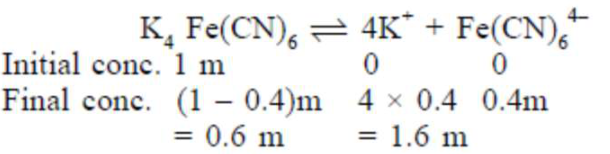# Solve the followingQuestion:

A 1 molal $\mathrm{K}_{4} \mathrm{Fe}(\mathrm{CN})_{6}$ solution has a degree of dissociation of $0.4$. Its boiling point is equal to that of another solution which contains $18.1$ weight percent of a non electrolytic solute $\mathrm{A}$. The molar mass of $A$ is___________ u. (Round off to the Nearest Integer).

$\left[\right.$ Density of water $\left.=1.0 \mathrm{~g} \mathrm{~cm}^{-3}\right]$

Solution:

(85)Effective molality $=0.6+1.6+0.4=2.6 \mathrm{~m}$

For same boiling point, the molality of another solution should also be

$2.6 \mathrm{~m}$. Now, $18.1$ weight percent solution means

18.1gm solute is present in $100 \mathrm{gm}$ solution and hence,

$(100-18.1=) 81.9 \mathrm{gm}$ water. Now, $2.6=\frac{18.1 / \mathrm{M}}{81.9 / 1000}$

$\therefore$ Molar mass of solute, $\mathrm{M}=85$